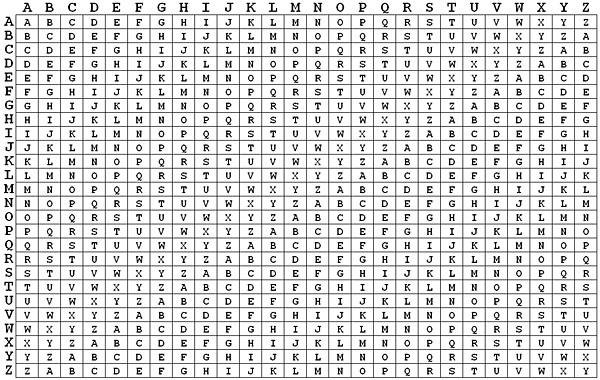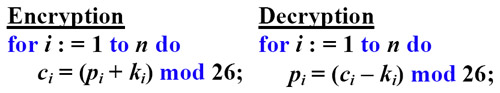# The Algebraic Nature of the Vigenère CipherWe discussed here that the alphabet is shifted to the left one position repeatedly to build the 26×26 Vigenère table. This is equivalent to shift the alphabet (i.e., the row heading of the Vigenère table) to the right one position at a time.For example, the row of B is obtained by shifting the row of A to the left one position. This is equivalent to shifting the alphabet to the right one position. For the row of B, A is shifted to B and B is shifted to C and, hence, A is encrypted to B and B is encrypted to C. Similarly, for the row of D which is three positions from A, A is shifted three positions to D, B is shifted three positions to E, and C is shifted three positions to F. Therefore, A, B and C are encrypted to D, E and F by shifting to the right three positions. In general, if a plaintext letter P is encrypted by a keyword letter K that is d positions from A, P is encrypted by K to the letter C that is d positions to the right of P. We have to take cyclic shifting into consideration. If the letters A, B, C, ..., Z are assigned the values of 0, 1, 2, ..., 25, each keyword letter is simply the distance from that letter to A. As a result, the ciphertext letter C is obtained as follows, where "mod" is the modulus arithmetic:

C = (P + d) mod 26

To sum it up, if the keyword is repeated enough number of times so that the total length is equal to the length of the plaintext, for plaintext p1p2...pn, keyword k1k2...kn and ciphertext c1c2...cn, we have

ci = (pi + ki) mod 26

Decryption is the reversed procedure by shifting the ciphertext to the left. Since shifting to the left is a subtraction, the decryption procedure is simply:

pi = (ci - ki) mod 26

With this in mind, it is very easy to program a Vigenère cipher as follows:An Important Note: In the ASCII code, letters A to Z are consecutive and K-'A' is the distance from A to the letter K. However, the 26 letters in the EBCDIC code are not consecutive. Therefore, it would be better to save the letters in an array of 26 elements and shift the array index rather than using K-'A'.